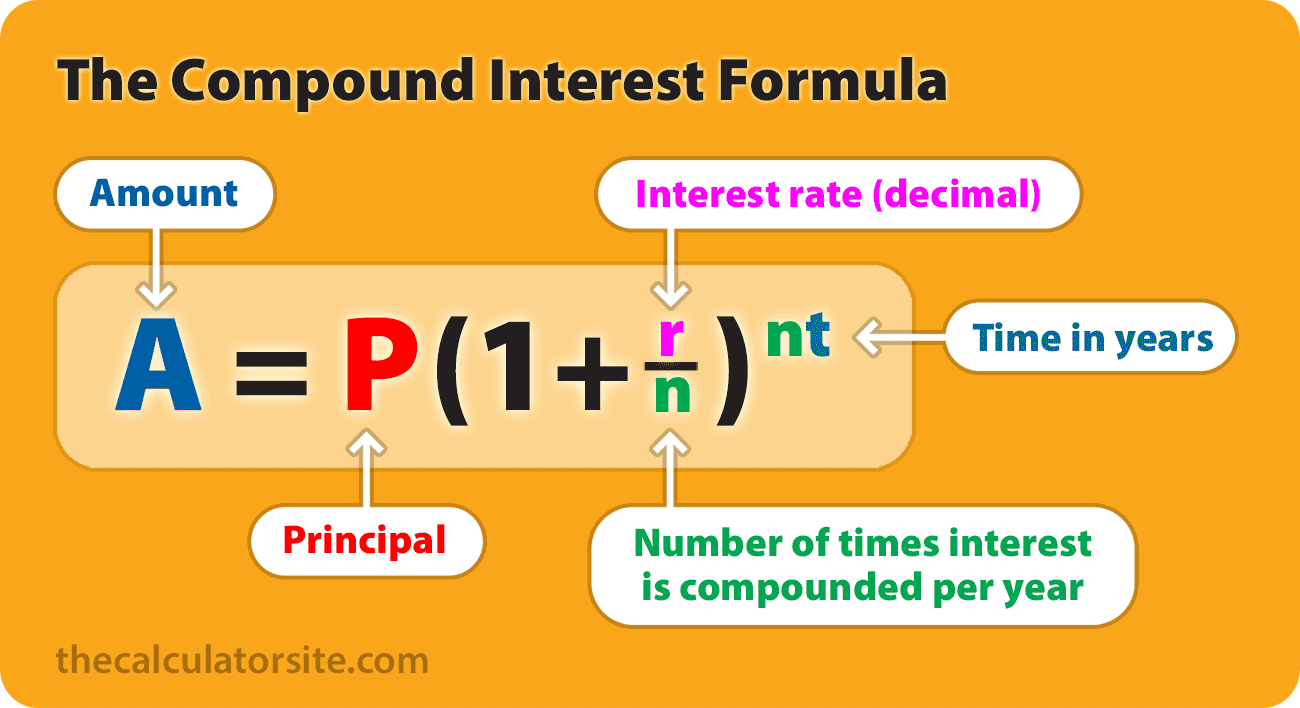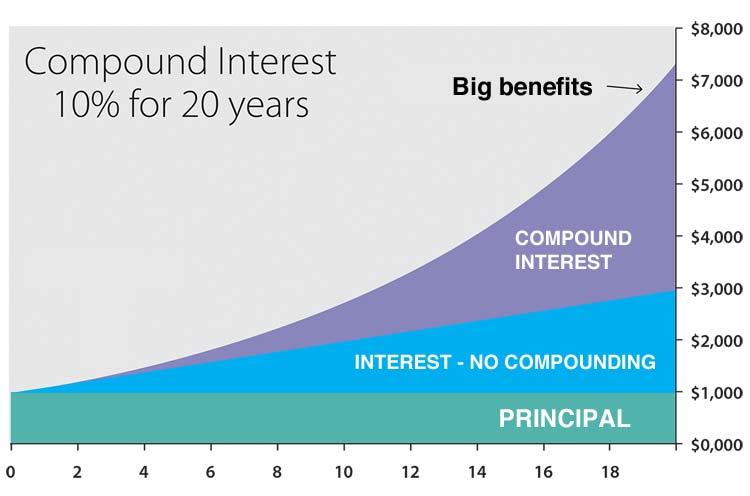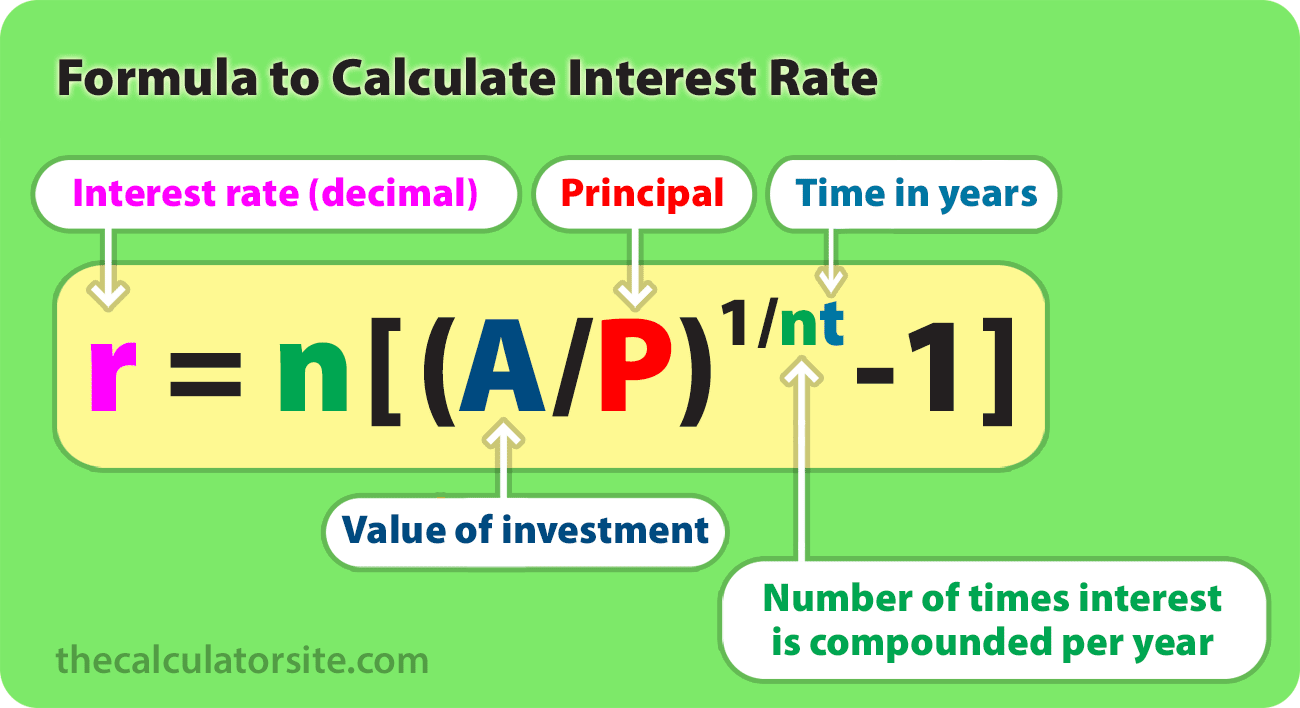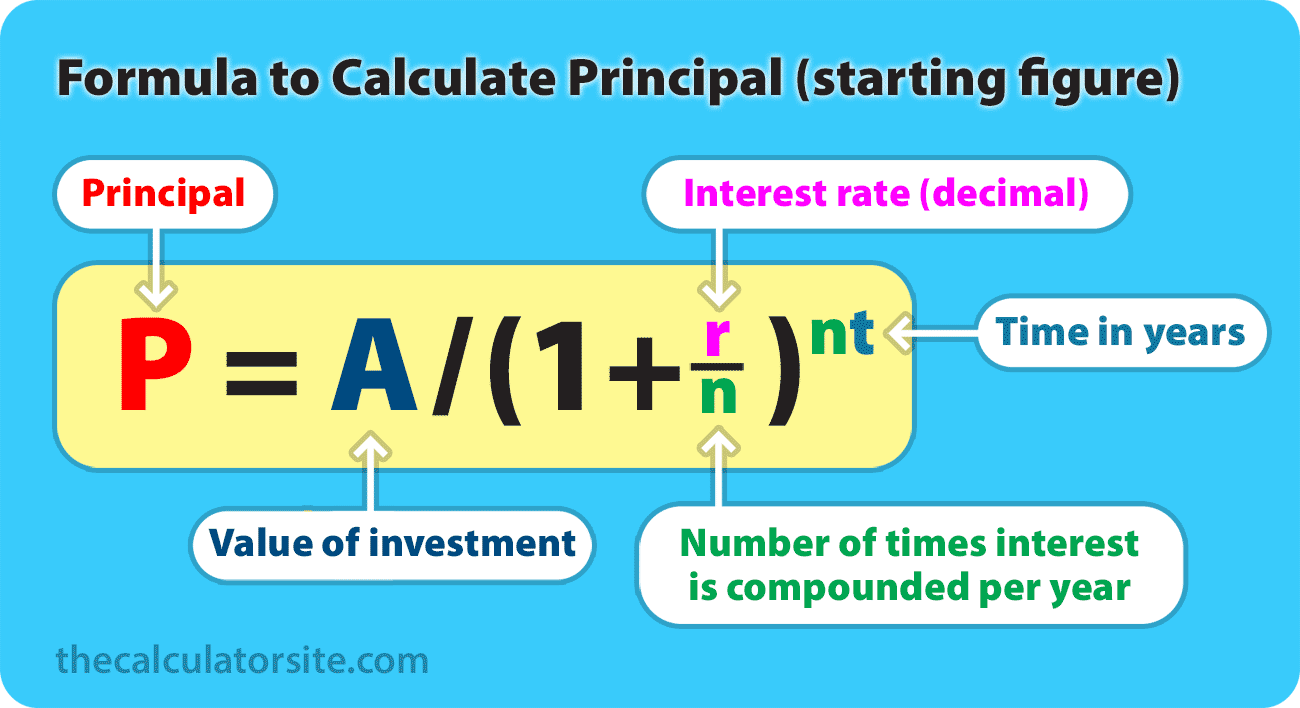# Compound Interest Formula With Examples

| Last update: 01 June 2022

Compound interest, or 'interest on interest', is calculated using the compound interest formula.

The formula for compound interest is A = P(1 + r/n)^nt, where P is the principal balance, r is the interest rate, n is the number of times interest is compounded per time period and t is the number of time periods.The concept of compound interest is that interest is added back to the principal sum so that interest is gained on that already-accumulated interest during the next compounding period. How important is it? Just ask Warren Buffett, one of the world's most successful investors:

"My wealth has come from a combination of living in America, some lucky genes, and compound interest."

In this article, we'll take a look at the compound interest formula in more depth. We'll also go through an example and discuss other variations of the formula that can help you to calculate the interest rate and time factor or to incorporate regular contributions. Should you wish to try some calculations using your own figures, we have a calculator for compound interest here.

## How to use the compound interest formula

To use the compound interest formula you will need figures for principal amount, annual interest rate, time factor and the number of compound periods. Once you have those, you can go through the process of calculating compound interest.

The formula for compound interest, including principal sum, is:
A = P(1 + r/n)^nt

Where:

• A = the future value of the investment/loan, including interest
• P = the principal investment amount (the initial deposit or loan amount)
• r = the annual interest rate (decimal)
• n = the number of times that interest is compounded per unit t
• t = the time the money is invested or borrowed for

It's worth noting that this formula gives you the future value of an investment or loan, which is compound interest plus the principal. Should you wish to calculate the compound interest only, you need to deduct the principal from the result. So, your formula looks like this:

Compounded interest only (without principal): P (1 + r/n)^nt - P

### Let's look at an example

If an amount of \$5,000 is deposited into a savings account at an annual interest rate of 5%, compounded monthly, the value of the investment after 10 years can be calculated as follows...

P = 5000.
r = 5/100 = 0.05 (decimal).
n = 12.
t = 10.

If we plug those figures into the formula, we get the following:

A = 5000 (1 + 0.05 / 12) (12 * 10) = 8235.05.

So, the investment balance after 10 years is \$8,235.05.

### Methodology

A few people have written to me asking me to explain step-by-step how we get the 8235.05. This all revolves around BODMAS / PEMDAS and the order of operations. Let's go through it:

A = 5000 (1 + 0.05 / 12) ^ (12(10))

(note that ^ means 'to the power of')

Using the order of operations we work out the totals in the brackets first. Within the first set of brackets, you need to do the division first and then the addition (division and multiplication should be carried out before addition and subtraction). We can also work out the 12(10). This gives us...

A = 5000 (1 + 0.00416) ^ 120

(note that the over-line in the calculation signifies a decimal that repeats to infinity. So, 0.00416666666...)

Then:

A = 5000 (1.00416) ^ 120

The exponent goes next. So, we calculate (1.00416) ^ 120.

This means we end up with:

5000 × 1.6470095042509848

= 8235.0475.

You may have seen some examples giving a formula of A = P ( 1+r ) t . This simplified formula assumes that interest is compounded once per period, rather than multiple times per period.

### The benefit of compound interest

I think it's worth taking a moment to examine the benefit of compound interest using our example. The benefit hopefully becomes clear when I tell you that without compound interest, your investment balance in the above example would be only \$7,500 (\$250 per year for 10 years, plus the original \$5000) by the end of the term. So, thanks to the wonder of compound interest, you stand to gain an additional \$735.05.

To give a graphical example, the graph below shows the result of \$1000 invested over 20 years at an interest rate of 10%. The principal figure is in green. The blue part of the graph shows the result of 10% interest without compounding. Finally, the purple part demonstrates the benefit of compound interest over those 20 years.## Interactive compound interest formula

I created the calculator below to show you the formula and resulting accrued investment/loan value (A) for the figures that you enter. Note that this calculator requires JavaScript to be enabled in your browser.

P ( 1 + r n ) ( n t ) = A

Note that we have a specialist calculator for compound interest with additional deposits and withdrawals relating to your savings and investments.

It may be that you want to manipulate the compound interest formula to work out the interest rate for IRR or CAGR, or a principal investment/loan figure. Here are the formulae you need.

This formula can help you work out the yearly interest rate you're getting on your savings, investment, personal loan or vehicle loan. Note that you should multiply your result by 100 to get a percentage figure (%)

## Formula for calculating interest rate (r)

r = n[(A/P)(1/nt)-1]

Where:

• r = the interest rate (decimal)
• A = the future value of the investment
• P = the principal investment amount
• n = the number of times that interest is compounded per unit t
• t = the time the money is invested for## Formula for calculating principal (P)

This formula is useful if you want to work backwards and find out how much you would need to start with in order to achieve a chosen future value.

P = A / (1 + r/n)nt

Where:

• P = the principal investment amount
• A = the future value of the investment
• r = the interest rate (decimal)
• n = the number of times that interest is compounded per unit t
• t = the time the money is invested forExample: Let's say your goal is to end up with \$10,000 in 5 years, and you can get an 8% interest rate on your savings, compounded monthly. Your calculation would be: P = 10000 / (1 + 0.08/12)(12×5) = \$6712.10. So, you would need to start off with \$6712.10 to achieve your goal.

## Formula for calculating time (t)

This variation of the formula works for calculating time (t), by using natural logarithms. You can see how this formula was worked out by reading this explanation on algebra.com.

t = ln(A/P) / n[ln(1 + r/n)]

Where:

• A = the value of the accrued investment/loan
• P = the principal amount
• r = the annual interest rate (decimal)
• n = the number of times that interest is compounded per unit t
• t = the time the money is invested or borrowed for

## Formula for regular contributions

A lot of people have asked me to include a single formula for compound interest with monthly additions. Believe me when I tell you that it isn't quite as simple as it sounds. In order to work out calculations involving monthly additions, you will need to use two formulae - our original one, listed above, plus the 'future value of a series' formula for the monthly additions.

At the request of readers, I've adapted the formula explanation to allow you to calculate periodic additions, not just monthly (added May 2016). These formulae assume that your frequency of compounding is the same as the periodic payment interval (monthly compounding, monthly contributions, etc). If you would like to try a version of the formula that allows you to have a different periodic payment interval to the compounding frequency, please see the ' periodic payments' section below.

If the additional deposits are made at the END of the period (end of month, year, etc), here are the two formulae you will need:

Compound interest for principal:

P(1+r/n)(nt)

Future value of a series:

PMT × {[(1 + r/n)(nt) - 1] / (r/n)}

If the additional deposits are made at the BEGINNING of the period (beginning of year, etc), here are the two formulae you will need:

Compound interest for principal:

P(1+r/n)(nt)

Future value of a series:

PMT × {[(1 + r/n)(nt) - 1] / (r/n)} × (1+r/n)

Where:

• A = the future value of the investment/loan, including interest
• P = the principal investment amount (the initial deposit or loan amount)
• PMT = the monthly payment
• r = the annual interest rate (decimal)
• n = the number of times that interest is compounded per unit t
• t = the time (months, years, etc) the money is invested or borrowed for

### Example

If an amount of \$5,000 is deposited into a savings account at an annual interest rate of 5%, compounded monthly, with additional deposits of \$100 per month (made at the end of each month). The value of the investment after 10 years can be calculated as follows...

P = 5000. PMT = 100. r = 5/100 = 0.05 (decimal). n = 12. t = 10.

If we plug those figures into the formulae, we get:

• Total = [ Compound interest for principal ] + [ Future value of a series ]
• Total = [ P(1+r/n)^(nt) ] + [ PMT × (((1 + r/n)^(nt) - 1) / (r/n)) ]
• Total = [ 5000 (1 + 0.05 / 12) ^ (12 × 10) ] + [ 100 × (((1 + 0.00416)^(12 × 10) - 1) / (0.00416)) ]
• Total = [ 5000 (1.00416) ^ (120) ] + [ 100 × (((1.00416^120) - 1) / 0.00416) ]
• Total = [ 8235.05 ] + [ 100 × (0.647009497690848 / 0.00416) ]
• Total = [ 8235.05 ] + [ 15528.23 ]
• Total = [ \$23,763.28 ]

So, the investment balance after 10 years is \$23,763.28.

## Different periodic payments

A few people have requested a version of the above formula that takes into account the number of periodic payments (both formulae above assume your periodic payments match the frequency of compounding). For example, your money may be compounded quarterly but you're making contributions monthly. In this case, you may wish to try this version of the formula, originally suggested by Darinth Douglas, and then expanded upon by Jean-Baptiste Delaroche. I'm most grateful for their input. This formula assumes that regular deposits are paid at the beginning rather than at the end of the period.

Compound interest for principal:

P(1+r/n)(nt)

Future value of a series:

PMT × p {[(1 + r/n)(nt) - 1] / (r/n)}

(With 'p' being the number of periodic payments in the compounding period, divided by n)

For more information about what to do with the formula when calendar intervals are irregular, see this useful page from Jon Wittwer.

### Example

An amount of \$100 is deposited quarterly into a savings account at an annual interest rate of 10%, compounded monthly. The value of the investment after 12 months can be calculated as follows...

PMT = 100. r = 0.1 (decimal). n = 12. p = 4/n = 4/12 = 0.3333333.

If we plug those figures into the formula, we get the following:

• Total = PMT × p {[(1 + r/n)(nt) - 1] / (r/n)}
• Total = 100 × 0.3333333 × {[(1 + 0.1 / 12) ^ (12 × 1) - 1] / (0.1 / 12)}
• Total = 100 × 0.3333333 × {[1.008333 ^ (12) - 1] / 0.008333}
• Total = 100 × 0.3333333 × {0.104709 / 0.008333}
• Total = 100 × 0.3333333 × 12.565583
• Total = 418.85

So, the investment balance after 12 months is \$418.85 (or \$418.84 if you round the numbers during the calculation).